# David T

•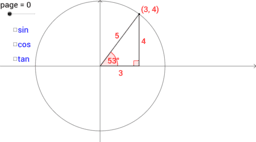### Trigonometry: CAST introduction

Activity

David T

•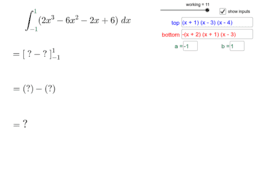### School Mathematics

Book

David T

•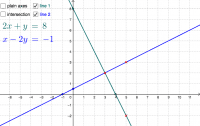### Simultaneous Equations: Graph move line

Activity

David T

•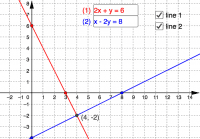### Simultaneous Equations: Graph input

Activity

David T

•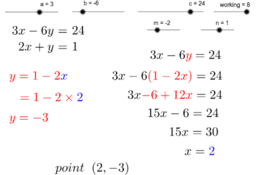### Simultaneous Equations:Substitution

Activity

David T

•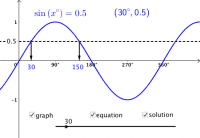### Trigonometry: equation solution graph 1 (sine)

Activity

David T

•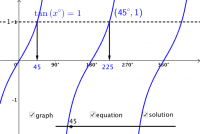### Trigonometry: equation solution graph 3 (tangent)

Activity

David T

•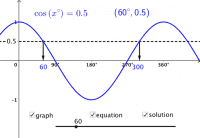### Trigonometry: equation solution graph 2 (cosine)

Activity

David T

•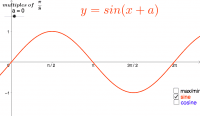### Trig Graph Transformation 2 (radians)

Activity

David T

•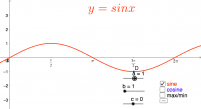### Trig Graph Transformation 3 (radians)

Activity

David T

•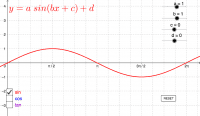### Trig Graph Transformation 4 (radians)

Activity

David T

•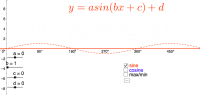### Trig Graph Transformation 4

Activity

David T

•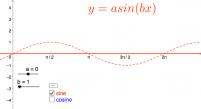### Trig Graph Transformation 1 (radians)

Activity

David T

•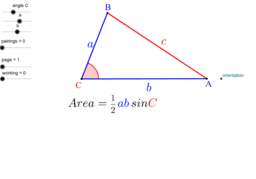### Trigonometry-Area Formula

Activity

David T

•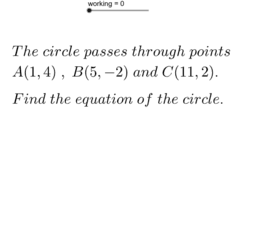### Equation of a Circle through 3 Points

Activity

David T

•### Simultaneous Equations:Elimination

Activity

David T

•### Standard Deviation Formulae

Activity

David T

•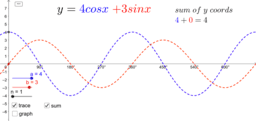### Wave Function

Activity

David T

•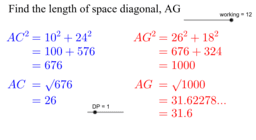### Pyth Thm: 3D cuboid

Activity

David T

•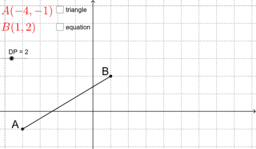### Pyth Thm: Coordinates

Activity

David T

•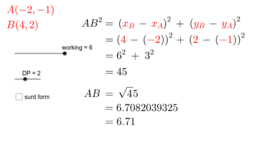### Distance Formula

Activity

David T

•### Equation of Tangent to a Circle

Activity

David T

•### Area between Curves

Activity

David T

•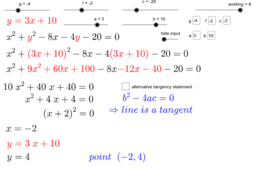Activity

David T